Some basic concepts in chemistry   Share

What is Some basic concepts in chemistry

'Some basic concepts of chemistry' is the most fundamental chapter of complete chemistry. It gives information about the atomic number and mass number of elements. In any chemical reaction, it is important for us to know about the number of reactants that will consume and the products that will produce, thus for estimating all these calculations, we use the laws of chemical combinations. In this chapter, there are other important topics as well as the mole concept, stoichiometry, molarity, normality, etc.

There are some important real life application based on the chapter of some basic concepts in chemistry.

• In cooking, it is always necessary to know the exact amount of ingredients to be add in the food to get the best and tasty dish. This calculation of ingredients in stoichiometry.• Saturn planet is much larger than earth but still, it is less dense. The specific gravity of Saturn is even less than 1.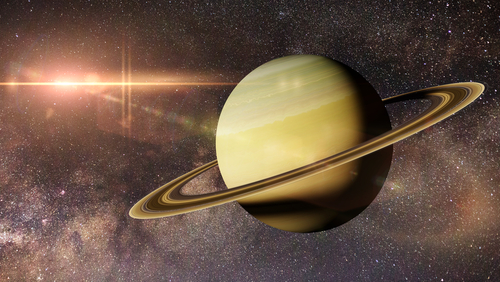• The GPS system that we use for finding out the way is related to stoichiometry. All these signals come from satellites. The stoichiometry helps to calculate the fuel and its components of reactions in the complete journey of satellites.Notes for Some Basic Concepts in Chemistry

In this section, you will study about the important topics of the chapter, overview, formulae and some important tips and guidelines for the preparation of the chapter at the best.

Important Topics

• Nature of Matter
• Laws of Chemical Combination
• Dalton's Atomic Theory
• Mole Concept
• Empirical Formula and Molecular Formula
• Stoichiometry and Stoichiometric Calculations
• Some Important Concentration Terms
Prepare Online for JEE Main/NEET

Crack JEE 2021 with JEE/NEET Online Preparation Program

Everything in the universe that has some mass and occupy some space is known as matter. Matter exists in three different physical forms i.e, solid, liquid and gas.

 Property Solid Liquid Gas Tightness Very tightly packed Tightly packed Loosely packed Intermolecular space Minimum Intermediate Maximum Force of attraction Maximum Intermediate Minimum Kinetic Energy Minimum Intermediate Maximum Density Maximum Intermediate Minimum Volume Fixed Fixed Variable Shape Fixed Variable Variable Compressibility factor Minimum Intermediate Maximum

At the macroscopic level, the matter can be classified into two categories i.e, mixtures and pure compounds as shown in the figure.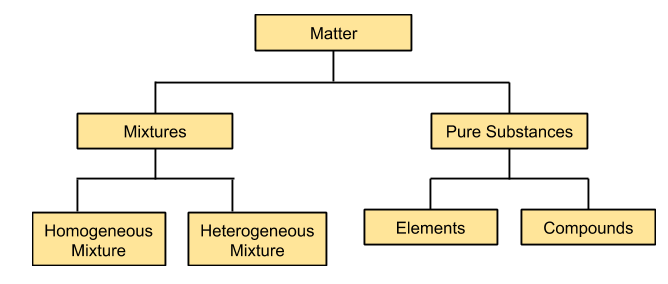Mixtures are those substances in which two or more components are mixed. Mixtures are further classified as homogeneous and heterogeneous mixtures. Homogeneous mixtures are the one in which components are present in a fixed ratio and the properties of this kind of mixture are the same throughout, for example, solution of sugar in water. But heterogeneous mixtures are those in which the components are not mixed in a definite ratio and properties of the mixture vary at different positions of the mixture, for example, sand in water.

Laws of Chemical Combination

The combination of elements to form some new product follows three basic laws as shown in the figure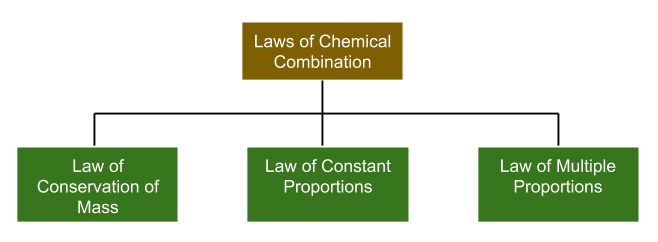• Law of conservation of mass: This law states that "matter can neither be created nor destroyed". This simply means that the number of reactants that are used in the reaction will be equal to the number of products formed.
• Law of definite proportions: This law states that any compound always contains its components in the fixed ratio by mass irrespective of its source and method of preparation.
• Law of multiple proportions: This law states that when two elements combine to form one or more than one compound, then the masses of one element that combine with the other element are in the ratio of small whole numbers.

Dalton's Atomic Theory

John Dalton proposed a theory about the matter and atoms in which he proposed the following postulates:

(i) Matter consists of small individual particles known as 'atoms'.
(ii) All atoms of any particular element have the same properties and same mass but atoms of different elements have different properties and mass.
(iii) Compounds are formed when atoms of different elements are combined in the fixed ratio.
(iv) Chemical reactions involve the reorganization of atoms.

Mole Concept

Mole is the standard unit to measure the number of particles like atoms or molecules in a given sample. Mathematically, one mole is equal to 6.022 x 1023. In other words, it can be said that it is equal to as many particles as there are atoms in 12g of the carbon-12 isotope. For example, if we have one mole of oxygen gas, that means we have 6.022 x 1023 molecules of O2.

Empirical Formula and Molecular formula

Empirical formula is the simplest whole-number ratio of atoms present in any particular molecule and the molecular formula is the actual representation of the number of atoms present in the molecule.

For example glucose i.e C6H12O6, its molecular formula is C6H12O6 but its empirical formula is CH2O.
Mathematically, the relation between empirical formula mass and molecular formula mass is given as follows:

where n is the simplest ratio.

Stoichiometry and Stoichiometric Calculations

This concept helps us to calculate the mass or amount of reactants and products in the given chemical reaction. For calculations, first we must have a balanced chemical equation, only then we can predict the mass of reactants and products. For example
The chemical reaction is given as follows:

Now, this chemical equation is a balanced equation, thus we are able to predict that one mole oxygen will combine with 2 moles of hydrogen and form 2 moles of water and thus accordingly, we can calculate the masses of the respective elements.

In this concept, we also study about the "limiting reagent". It is the substance that is present in reactants in a smaller amount. In other words, limiting reagent is a substance which will be completely finished or react in the given chemical reaction.

Some important Concentration Terms

• Mole fraction: It is the ratio of the moles of any substance present in the solution to the total moles of the solution. Mathematically, it is given as follows:

where nA is the moles of component A and nB is the moles of component B.
• Molarity: It is the concentration of any substance present in the solution. In other words, it is moles of solute per unit volume of solution in litres. Mathematically, it can be given as follows:

• Molality: It is the moles of solute dissolved in the given amount of solvent. Mathematically, it can be represented as follows:

• Normality: It is defined as the number of equivalents of solute dissolved in one litre volume of solution. Mathematically, it can be represented as follows:

How to prepare for States of matter?

• This chapter is the beginning of chemistry. This chapter is one of the most important chapters of the complete chemistry syllabus. Its concepts, laws, numerical and graphs all are important both for basic foundation of chemistry and for scoring good marks in the examination.

• Read this chapter carefully, as it has all the basic concepts like the mole concept, stoichiometry, molarity, normality, etc.

• This chapter is the foundation stone of the whole of the chemistry syllabus.

• Rest this chapter is very simple, just be regular and be consistent in your numerical practice.

Chemistry Chapter- wise Notes for Engineering and Medical Exams

 Chapters No. Chapters Name Chapter 2 States of matter Chapter 3 Atomic Structure Chapter 4 Solutions Chapter 5 Chemical Thermodynamics Chapter 6 Equilibrium Chapter 7 Redox Reaction and Electrochemistry Chapter 8 Chemical kinetics Chapter 9 Surface Chemistry Chapter 10 General Principle and processes of Isolation of metals Chapter 11 Classification of Elements and Periodic table Chapter 12 Hydrogen Chapter 13 p- block Elements Chapter 14 s-block Elements (Alkali and Alkaline Earth Metals) Chapter 15 Chemical Bonding and Molecular Structure Chapter 16 d- and f- BLOCK ELEMENTS Chapter 17 Coordination Compounds Chapter  18 Environmental Chemistry Chapter 19 Purification and Characterisation of Organic Compounds Chapter 20 Some Basic Principles of Organic Chemistry Chapter 21 Hydrocarbons Chapter 22 Organic Compounds containing Halogens Chapter 23 Organic Compounds containing Oxygen Chapter 24 Organic Compounds Containing Nitrogen Chapter 25 Polymers Chapter 26 Biomolecules Chapter 27 Chemistry in Everyday Life Chapter 28 Principles Related to Practical Chemistry

Topics from Some basic concepts in chemistry

• Precision and accuracy, significant figures, S.I. Units, dimensional analysis ( JEE Main, KCET, MHT-CET ) (18 concepts)
• Concept of atom, molecule, element and compund ( JEE Main, KCET, MHT-CET ) (10 concepts)
• Physical quantities and their measurements in chemistry ( JEE Main, KCET, MHT-CET ) (7 concepts)
• Percentage composition, Empirical and molecular formulae ( JEE Main, KCET, MHT-CET ) (4 concepts)
• Chemical equations and stiochiometry ( JEE Main, KCET, MHT-CET ) (13 concepts)
• Atomic and molecular masses, mole concept, molar mass ( JEE Main, KCET, MHT-CET ) (10 concepts)
• Matter and its nature ( JEE Main, KCET, MHT-CET ) (4 concepts)
• Dalton's atomic theory ( JEE Main, KCET, MHT-CET ) (8 concepts)
• Laws of chemical combination ( JEE Main, KCET, MHT-CET ) (8 concepts)
• Importance of chemistry and Nature of matter ( JEE Main, KCET, MHT-CET ) (6 concepts)
• Stoichiometry, Stoichiometric Calculations ( JEE Main, KCET, MHT-CET ) (11 concepts)
• Uncertainty in measurement ( JEE Main, KCET, MHT-CET ) (3 concepts)
• Fundamental Laws of Chemical combinations ( JEE Main, KCET, MHT-CET ) (3 concepts)
• Percentage Composition ( JEE Main, KCET, MHT-CET ) (6 concepts)
• Dalton’s Atomic Model ( JEE Main, KCET, MHT-CET ) (3 concepts)
• Atomic And Molecular Masses ( JEE Main, KCET, MHT-CET ) (3 concepts)
• Mole Concept And Molar Masses ( JEE Main, KCET, MHT-CET ) (3 concepts)
• Smart Tips: Some Basic Concept in Chemistry ( JEE Main, KCET, MHT-CET ) (12 concepts)
• Atomic Models ( JEE Main, SRMJEEE, TS EAMCET, MET, AP EAMCET, AP EAMCET Pharmacy, TS EAMCET Pharmacy, BITSAT Pharmacy, GUJCET Pharmacy ) (3 concepts)
• Developments leading to the Bohr’s model of atom ( JEE Main, SRMJEEE, TS EAMCET, MET, AP EAMCET, AP EAMCET Pharmacy, TS EAMCET Pharmacy, BITSAT Pharmacy, GUJCET Pharmacy ) (1 concepts)
• Particle Nature of electromagnetic radiation ( JEE Main, SRMJEEE, TS EAMCET, MET, AP EAMCET, AP EAMCET Pharmacy, TS EAMCET Pharmacy, BITSAT Pharmacy, GUJCET Pharmacy ) (1 concepts)
• Photoelectric Effect ( JEE Main, SRMJEEE, TS EAMCET, MET, AP EAMCET, AP EAMCET Pharmacy, TS EAMCET Pharmacy, BITSAT Pharmacy, GUJCET Pharmacy ) (1 concepts)
• Line Spectrum of hydrogen ( JEE Main, SRMJEEE, TS EAMCET, MET, AP EAMCET, AP EAMCET Pharmacy, TS EAMCET Pharmacy, BITSAT Pharmacy, GUJCET Pharmacy ) (1 concepts)
• Bohr model for hydrogen atom ( JEE Main, SRMJEEE, TS EAMCET, MET, AP EAMCET, AP EAMCET Pharmacy, TS EAMCET Pharmacy, BITSAT Pharmacy, GUJCET Pharmacy ) (3 concepts)
• Dual behavior of matter ( JEE Main, SRMJEEE, TS EAMCET, MET, AP EAMCET, AP EAMCET Pharmacy, TS EAMCET Pharmacy, BITSAT Pharmacy, GUJCET Pharmacy ) (1 concepts)
• Heisenberg’s uncertainty principle ( JEE Main, SRMJEEE, TS EAMCET, MET, AP EAMCET, AP EAMCET Pharmacy, TS EAMCET Pharmacy, BITSAT Pharmacy, GUJCET Pharmacy ) (1 concepts)
• Elementary ideas of quantum mechanics ( JEE Main, SRMJEEE, TS EAMCET, MET, AP EAMCET, AP EAMCET Pharmacy, TS EAMCET Pharmacy, BITSAT Pharmacy, GUJCET Pharmacy ) (1 concepts)
• Quantum mechanical model of atom ( JEE Main, SRMJEEE, TS EAMCET, MET, AP EAMCET, AP EAMCET Pharmacy, TS EAMCET Pharmacy, BITSAT Pharmacy, GUJCET Pharmacy ) (6 concepts)
• Smart tips: Classification of Elements and Periodic Table ( JEE Main, COMEDK UGET ) (1 concepts)
• Genesis of periodic classification ( JEE Main, COMEDK UGET ) (1 concepts)
• Modern periodic law and the present form of the periodic table ( JEE Main, COMEDK UGET ) (3 concepts)
• Nomenclature of elements with atomic numbers >100 ( JEE Main, COMEDK UGET ) (1 concepts)
• Electronic configurations and types of elements:s-, p-, d-, f-, blocks ( JEE Main, COMEDK UGET ) (5 concepts)
• Periodic trends in properties of elements ( JEE Main, COMEDK UGET ) (8 concepts)
• Modem periodic law ( JEE Main, COMEDK UGET ) (1 concepts)
• Volume Strength of H2O2 Solution and Hardness of Water ( JEE Main, KCET, MHT-CET ) (2 concepts)
• n-Factor or Valence Factor ( JEE Main, KCET, MHT-CET ) (2 concepts)

Important Books for Some basic concepts in chemistry

•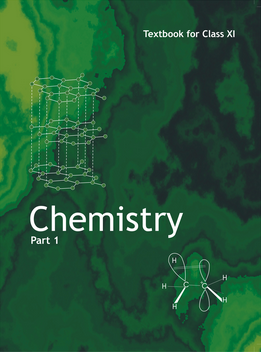•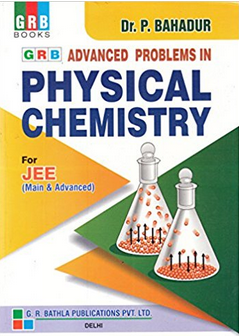Exams
Articles
Questions